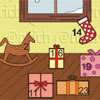Search by Topic

Resources tagged with Interactivities similar to The Set of Numbers:

Filter by: Content type:
Age range:
Challenge level:

There are 197 results

Broad Topics > Information and Communications Technology > Interactivities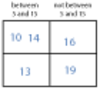More Carroll Diagrams

Age 7 to 11 Challenge Level:

How have the numbers been placed in this Carroll diagram? Which labels would you put on each row and column?One Million to Seven

Age 7 to 11 Challenge Level:

Start by putting one million (1 000 000) into the display of your calculator. Can you reduce this to 7 using just the 7 key and add, subtract, multiply, divide and equals as many times as you like?Number Differences

Age 7 to 11 Challenge Level:

Place the numbers from 1 to 9 in the squares below so that the difference between joined squares is odd. How many different ways can you do this?Domino Numbers

Age 7 to 11 Challenge Level:

Can you see why 2 by 2 could be 5? Can you predict what 2 by 10 will be?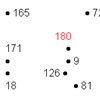A Dotty Problem

Age 7 to 11 Challenge Level:

Starting with the number 180, take away 9 again and again, joining up the dots as you go. Watch out - don't join all the dots!Light the Lights

Age 5 to 7 Challenge Level:

Investigate which numbers make these lights come on. What is the smallest number you can find that lights up all the lights?Countdown

Age 7 to 14 Challenge Level:

Here is a chance to play a version of the classic Countdown Game.Carroll Diagrams

Age 5 to 11 Challenge Level:

Use the interactivities to fill in these Carroll diagrams. How do you know where to place the numbers?Growing Garlic

Age 5 to 7 Challenge Level:

Ben and his mum are planting garlic. Use the interactivity to help you find out how many cloves of garlic they might have had.Coded Hundred Square

Age 7 to 11 Challenge Level:

This 100 square jigsaw is written in code. It starts with 1 and ends with 100. Can you build it up?Seven Flipped

Age 7 to 11 Challenge Level:

Investigate the smallest number of moves it takes to turn these mats upside-down if you can only turn exactly three at a time.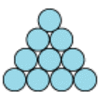Difference

Age 7 to 11 Challenge Level:

Place the numbers 1 to 10 in the circles so that each number is the difference between the two numbers just below it.Venn Diagrams

Age 5 to 11 Challenge Level:

Use the interactivities to complete these Venn diagrams.Age 5 to 7 Challenge Level:

Twenty four games for the run-up to Christmas.Age 7 to 11 Challenge Level:

If you have only four weights, where could you place them in order to balance this equaliser?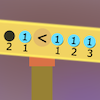Number Balance

Age 5 to 7 Challenge Level:

Can you hang weights in the right place to make the equaliser balance?Making Trains

Age 5 to 7 Challenge Level:

Can you make a train the same length as Laura's but using three differently coloured rods? Is there only one way of doing it?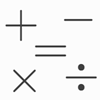Which Symbol?

Age 7 to 11 Challenge Level:

Choose a symbol to put into the number sentence.I Like ...

Age 5 to 7 Challenge Level:

Mr Gilderdale is playing a game with his class. What rule might he have chosen? How would you test your idea?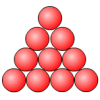Change Around

Age 5 to 7 Challenge Level:

Move just three of the circles so that the triangle faces in the opposite direction.Got it Article

Age 7 to 14

This article gives you a few ideas for understanding the Got It! game and how you might find a winning strategy.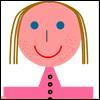The Brown Family

Age 5 to 7 Challenge Level:

Use the information about Sally and her brother to find out how many children there are in the Brown family.Factor Lines

Age 7 to 14 Challenge Level:

Arrange the four number cards on the grid, according to the rules, to make a diagonal, vertical or horizontal line.Ring a Ring of Numbers

Age 5 to 7 Challenge Level:

Choose four of the numbers from 1 to 9 to put in the squares so that the differences between joined squares are odd.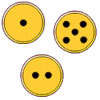Fair Exchange

Age 5 to 7 Challenge Level:

In your bank, you have three types of coins. The number of spots shows how much they are worth. Can you choose coins to exchange with the groups given to make the same total?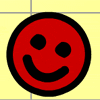Complete the Square

Age 5 to 7 Challenge Level:

Complete the squares - but be warned some are trickier than they look!Code Breaker

Age 7 to 11 Challenge Level:

This problem is based on a code using two different prime numbers less than 10. You'll need to multiply them together and shift the alphabet forwards by the result. Can you decipher the code?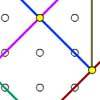Coordinate Tan

Age 7 to 11 Challenge Level:

What are the coordinates of the coloured dots that mark out the tangram? Try changing the position of the origin. What happens to the coordinates now?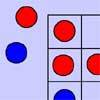Red Even

Age 7 to 11 Challenge Level:

You have 4 red and 5 blue counters. How many ways can they be placed on a 3 by 3 grid so that all the rows columns and diagonals have an even number of red counters?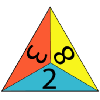One Big Triangle

Age 5 to 7 Challenge Level:

Make one big triangle so the numbers that touch on the small triangles add to 10.Teddy Town

Age 5 to 14 Challenge Level:

There are nine teddies in Teddy Town - three red, three blue and three yellow. There are also nine houses, three of each colour. Can you put them on the map of Teddy Town according to the rules?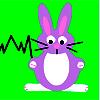Rabbit Run

Age 7 to 11 Challenge Level:

Ahmed has some wooden planks to use for three sides of a rabbit run against the shed. What quadrilaterals would he be able to make with the planks of different lengths?Share Bears

Age 5 to 7 Challenge Level:

Yasmin and Zach have some bears to share. Which numbers of bears can they share so that there are none left over?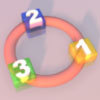More Numbers in the Ring

Age 5 to 7 Challenge Level:

If there are 3 squares in the ring, can you place three different numbers in them so that their differences are odd? Try with different numbers of squares around the ring. What do you notice?Arrangements

Age 7 to 11 Challenge Level:

Is it possible to place 2 counters on the 3 by 3 grid so that there is an even number of counters in every row and every column? How about if you have 3 counters or 4 counters or....?Multiplication Square Jigsaw

Age 7 to 11 Challenge Level:

Can you complete this jigsaw of the multiplication square?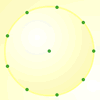Round Peg Board

Age 5 to 11 Challenge Level:

A generic circular pegboard resource.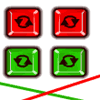Right Angle Challenge

Age 5 to 7 Challenge Level:

How many right angles can you make using two sticks?Same Length Trains

Age 5 to 7 Challenge Level:

How many trains can you make which are the same length as Matt's, using rods that are identical?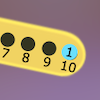Are You Well Balanced?

Age 5 to 7 Challenge Level:

Can you work out how to balance this equaliser? You can put more than one weight on a hook.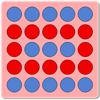Spot Thirteen

Age 7 to 11 Challenge Level:

Choose 13 spots on the grid. Can you work out the scoring system? What is the maximum possible score?Find the Difference

Age 5 to 7 Challenge Level:

Place the numbers 1 to 6 in the circles so that each number is the difference between the two numbers just below it.Sorting Numbers

Age 5 to 7 Challenge Level:

Use the interactivity to sort these numbers into sets. Can you give each set a name?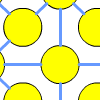One to Fifteen

Age 7 to 11 Challenge Level:

Can you put the numbers from 1 to 15 on the circles so that no consecutive numbers lie anywhere along a continuous straight line?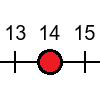Tug of War

Age 5 to 7 Challenge Level:

Can you use the numbers on the dice to reach your end of the number line before your partner beats you?Junior Frogs

Age 5 to 11 Challenge Level:

Have a go at this well-known challenge. Can you swap the frogs and toads in as few slides and jumps as possible?A Square of Numbers

Age 7 to 11 Challenge Level:

Can you put the numbers 1 to 8 into the circles so that the four calculations are correct?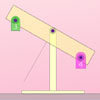Weighted Numbers

Age 5 to 7 Challenge Level:

Use the number weights to find different ways of balancing the equaliser.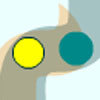Tubular Path

Age 7 to 11 Challenge Level:

Can you make the green spot travel through the tube by moving the yellow spot? Could you draw a tube that both spots would follow?